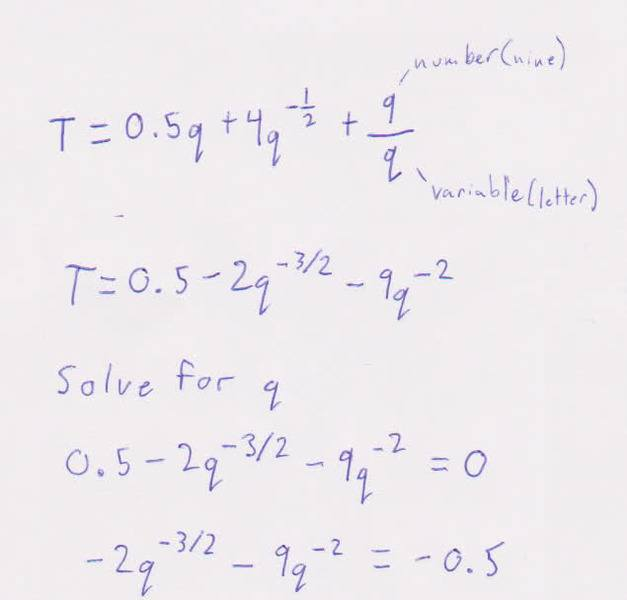# How to solve for q after finding derivative (derived) equation?

noid
I have started with the formula.

T = 0.5q+4q^(-1/2)+9/q

I have derived and am trying to solve for q but have gotten caught up on how to solve when I have to subtract two variables with different base numbers and different exponents.Any ideas?

Last edited:

Homework Helper
I have started with the formula.

T = 0.5q+4q^(-1/2)+9/q

I have derived and am trying to solve for q but have gotten caught up on how to solve when I have to subtract two variables with different base numbers and different exponents.Any ideas?

For one thing you made a mistake to start. If you divide both sides by q then the left side should be T/q. The correct way to go is probably to set q^(-1/2)=w, then express the other powers of q in terms w. If you work it through you'll get a quartic equation in w, a polynomial equation with w^4 as the highest power. The good news is that in principle, has a analytic solution. The bad news is that it's so complex as to to be basically useless. Are you sure you haven't made a mistake in deriving the equation you showed us? Where did it come from??

noid
Sorry for the misunderstanding: The second line is actually the derivative in respect to q of the first line (I forgot to put ' after T). Then from the second line I want to find what q is (after setting T to 0).